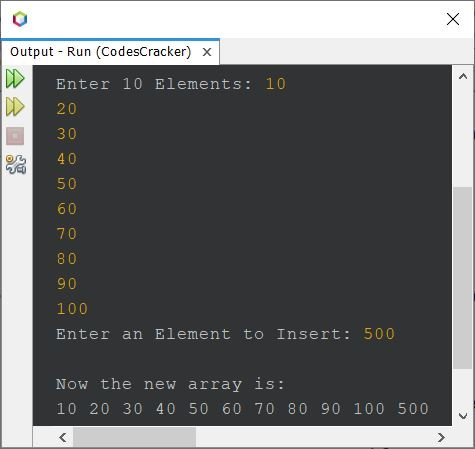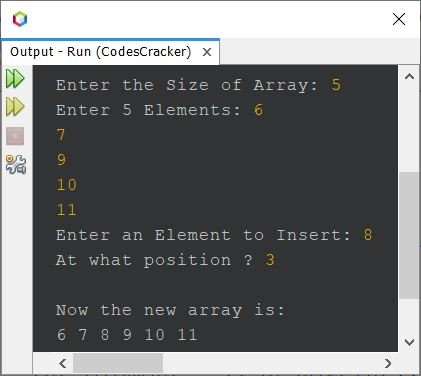# Java Program to Insert an Element in an Array

This article covers multiple programs in Java that inserts an element in an array, entered by user at run-time of the program. Here are the list of programs covered by this article:

• Insert an element at the end of an array of 10 elements
• Insert an element at the end of an array of n elements
• Insert an element in an array at a given position

## Insert an Element at the End of an Array in Java

The question is, write a Java program to insert an element in an array at the end. The program given below is its answer:

```import java.util.Scanner;

public class CodesCracker
{
public static void main(String[] args)
{
int i, element;
int[] arr = new int;
Scanner scan = new Scanner(System.in);

System.out.print("Enter 10 Elements: ");
for(i=0; i<10; i++)
arr[i] = scan.nextInt();

System.out.print("Enter an Element to Insert: ");
element = scan.nextInt();
arr[i] = element;

System.out.println("\nNow the new array is: ");
for(i=0; i<11; i++)
System.out.print(arr[i]+ " ");
}
}```

The snapshot given below shows the sample run of above program, with user input 10, 20, 30, 40, 50, 60, 70, 80, 90, 100 as ten elements and 500 as new element to insert at the end of array:#### Modified Version of Previous Program

Since previous program works with 10 elements only. Therefore after modifying that program, the program given below allows user to define the size of array too, along with its elements:

```import java.util.Scanner;

public class CodesCracker
{
public static void main(String[] args)
{
int i, element, n;
Scanner scan = new Scanner(System.in);

System.out.print("Enter the Size of Array: ");
n = scan.nextInt();
int[] arr = new int[n+1];
System.out.print("Enter " +n+ " Elements: ");
for(i=0; i<n; i++)
arr[i] = scan.nextInt();

System.out.print("Enter an Element to Insert: ");
element = scan.nextInt();
arr[i] = element;

System.out.println("\nNow the new array is: ");
for(i=0; i<(n+1); i++)
System.out.print(arr[i]+ " ");
}
}```

The sample run with user input 5 as size, 11, 12, 13, 14, 15 as five elements, and 16 as new element to insert, is shown in the snapshot given below:## Insert an Element in an Array at a Given Position in Java

This is the last program of this article, created to insert an element in an array at any specified (given) position:

```import java.util.Scanner;

public class CodesCracker
{
public static void main(String[] args)
{
int i, tot;
Scanner scan = new Scanner(System.in);

System.out.print("Enter the Size of Array: ");
int n = scan.nextInt();
int[] arr = new int[n+1];
System.out.print("Enter " +n+ " Elements: ");
for(i=0; i<n; i++)
arr[i] = scan.nextInt();

System.out.print("Enter an Element to Insert: ");
int element = scan.nextInt();
System.out.print("At what position ? ");
int pos = scan.nextInt();

if(pos<n)
{
for(i=n; i>=pos; i--)
arr[i] = arr[i-1];
arr[i] = element;
System.out.println("\nNow the new array is: ");
for(i=0; i<(n+1); i++)
System.out.print(arr[i]+ " ");
}
else
System.out.println("\nInvalid Input!");
}
}```

The sample run with user input 5 as size, 6, 7, 9, 10, 11 as five elements, 8 as new element to insert, and 3 as position to insert the element, is given below:#### Same Program in Other Languages

Java Online Test

« Previous Program Next Program »